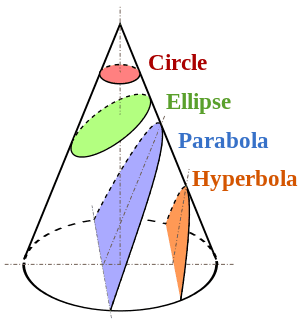# How can you tell what kind of orbit a body will have?

• 21joanna12

#### 21joanna12

Is there a way of telling whether the orbit of a body around another, or rather of both around their centre of mass, will give the object in question a circular, elliptical, hyperbolic or parabolic orbit?

Thank you!

If you know the objects' current velocity, you can calculate the trajectory/orbit.

On a circular orbit, the speed is, for one, always at a right angle to radius. For another, it has a specific value. For a test body in the field of a point primary, since the centrifugal acceleration is vˇ2/R and gravitational acceleration is GM/Rˇ2, it means that the speed has to be the specific value of square root of (GM/R).

1. So the orbit is circular if the speed is exactly square root of (GM/R) and exactly at the right angle to radius.
2. If the speed is exactly square root of (2GM/R) then the orbit is parabolic unless v is collinear with R, in which case it is a straight line (and goes to infinity if it is away from primary)
3. If the speed is any value bigger than square root of (2GM/R) then the orbit is hyperbolic unless v is collinear with R, in which case it also is a straight line and goes to infinity if it is away from primary.
4. If the speed is any value less than square root of (2GM/R) but over zero then the orbit is elliptical except in two special cases - in case it is collinear with R, in which case it is a segment of straight line that does not go to infinity if it is away from primary, and the other special case stated in point 1), of the speed being both exactly square root of (GM/R) as well as exactly right angle to radius
5. If the speed is zero then the orbit is the radius.

On a circular orbit, the speed is, for one, always at a right angle to radius. For another, it has a specific value. For a test body in the field of a point primary, since the centrifugal acceleration is vˇ2/R and gravitational acceleration is GM/Rˇ2, it means that the speed has to be the specific value of square root of (GM/R).

1. So the orbit is circular if the speed is exactly square root of (GM/R) and exactly at the right angle to radius.
2. If the speed is exactly square root of (2GM/R) then the orbit is parabolic unless v is collinear with R, in which case it is a straight line (and goes to infinity if it is away from primary)
3. If the speed is any value bigger than square root of (2GM/R) then the orbit is hyperbolic unless v is collinear with R, in which case it also is a straight line and goes to infinity if it is away from primary.
4. If the speed is any value less than square root of (2GM/R) but over zero then the orbit is elliptical except in two special cases - in case it is collinear with R, in which case it is a segment of straight line that does not go to infinity if it is away from primary, and the other special case stated in point 1), of the speed being both exactly square root of (GM/R) as well as exactly right angle to radius
5. If the speed is zero then the orbit is the radius.
Thank you so mch for this! I was wondering if you have a link to derivations of these results?

Bump! Does anyone have a derivation for the results above?

Write down the possible velocities like so:
##0<V_c<V_e<\infty##
which corresponds to:
an ellipse for ##V## between ##0## and ##V_e## with the special case of a circle at ##V_c##, a parabola for ##V_e## and a hyperbola for anything larger.

You wrote the conditions for ##V_c## yourself in your recent thread about binaries. Just assume M>>m and you'll get the equation snorkack wrote.
The case of parabola is the case of V being equal to escape velocity. It's easily derived from conservation of energy:
http://en.wikipedia.org/wiki/Escape_velocity

As for why such progression can be assumed:
Orbits are conical sections as shown here:where the difference between them can be reduced purely to eccentricity.
As can be seen here:
http://en.wikipedia.org/wiki/Orbital_eccentricity#Definition
here:
http://en.wikipedia.org/wiki/Laplace–Runge–Lenz_vector#Derivation_of_the_Kepler_orbits
or here:
http://en.wikipedia.org/wiki/Kepler_problem#Solution_of_the_Kepler_problem
##e=\sqrt{1+\frac{2EL^2}{k^2m}}##
the eccentricity is a function of orbital energy and angular momentum. But since the latter is squared, the sign of total energy E determines whether eccentricity ever goes below 1.

When total energy is less than 0 (KE < PE) the result is eccentricity of less than 1 (ellipse), if total energy
equals 0 (KE=PE) the eccentricity is 1 (parabola), and if it's more than 0 (KE>PE) the result is a hyperbola.
The circle is here a special case of an ellipse.

For a given distance from the central body (i.e., equal PE) the sign of total E is dependent only on the value of KE (i.e., velocity).

•